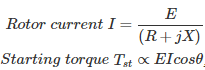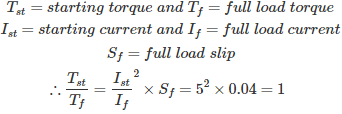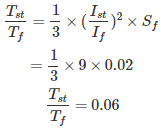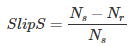# MCQs on Electrical Machines

##### Page 32 of 114. Go to page 1 2 3 4 5 6 7 8 9 10 11 12 13 14 15 16 17 18 19 20 21 22 23 24 25 26 27 28 29 30 31 32 33 34 35 36 37 38 39 40 41 42 43 44 45 46 47 48 49 50 51 52 53 54 55 56 57 58 59 60 61 62 63 64 65 66 67 68 69 70 71 72 73 74 75 76 77 78 79 80 81 82 83 84 85 86 87 88 89 90 91 92 93 94 95 96 97 98 99 100 101 102 103 104 105 106 107 108 109 110 111 112 113 114
01․ Blocked rotor test is used to find which of the following losses?
constant losses
variable losses
both 1 and 2
rotational loss

In blocked rotor test, applied voltage is very small, so the flux is very small. Therefore we can neglect the stator core loss. As the rotor is blocked, there is no rotation. So rotational losses are zero. Rotor core losses can also be neglected because rotor frequency is too small. Therefore the watt-meter reading is considered to be stator and rotor full load copper loss which are variable losses.

02․ Which of the following are the starting methods for squirrel cage induction motor?
Auto transformer starting
Stator resistance starting
Star-delta starting
All of the above

As the rotor of induction motor is under short circuit, a large voltage induced in it during starting produces a high rotor current which comes through the stator. Therefore the motor draws large current at starting. To control the rotor current in squirrel cage motor only option is to go for reduced voltage starting. By using all the above methods we can get the reduced voltage at starting. These above methods are used as there is no rotor conductor but rotor bars only.

03․ Which of the following is the best way of starting for slip ring induction motor?
Auto transformer starting
Stator resistance starting
Rotor resistance starting
Star-delta startingThe resistance of rotor can be adjusted to a suitable value which gives a high starting torque besides controlling the rotor current as well as stator current. The decrease in the current is dominated by increase in the power factor, as well as rated voltage applied across the stator which produces high staring torque. Technically this is the best way of starting slip-ring induction motor, because starting torque is high with low starting current.

04․ An induction motor has a full load slip of 0.04. Its starting current is 5 times its full load current. what is the ratio of starting torque to the full load torque?
0.2
0.5
1
1005․ A starting torque of 40 Nm is developed in an induction motor by an auto transformer starter with a tapping of 30%. If the tapping of auto transformer is 60%, then what is the starting torque?
160 N-m
100 N-m
240 N-m
80 N-m06․ Which of the following statements is/are correct?
High frequency power supplies are light weight
Transformer size get reduced at high frequency
Both 1 and 2
None of the above

From the induced emf equation of transformer E ∝ φf For same emf, φf = constant φ1f1 = φ2f2 B1A1f1 = B2A2f2 For constant flux density B1 = B2 A1f1 = A2f2 For high frequency f2 > f1 A2 < A1 Therefore at high frequencies transformer size get reduced and also light weight.

07․ The advantage of the double squirrel cage induction motor over single cage rotor is that its
slip is larger.
efficiency is higher.
starting current is lower.
power factor is higher.

The outer cage has high resistance and low reactance while the inner cage has low resistance and high reactance. Therefore at starting, the current is mainly confined to the outer cage with a consequent decrease in starting current and an increase in starting torque. By increasing the starting resistance, the starting torque is increased and by increasing starting reactance running torque is improved.

08․ An induction motor is started using auto transformer and it has a full load slip(Sf) of 0.02. Its starting current(Ist) is 3 times its full load current(If). What is the ratio of starting torque(Tst) to full load torque(Tf)?
0.06
0.6
0.18
0.3

The motor is started using auto transformer. The ratio of Tst to Tf is09․ In induction generator the slip is always
positive.
negative.
zero.
any of the above.

An Induction motor acts as a generator when its speed increases than the speed of its stator rotating magnetic field (RMF). If a prime over is connected to the shaft and the stator is connected to ac supply and it is rotated at a speed greater than speed of RMF, so slip becomes negative and torque becomes negative along with rotor current and power.If Nr > Ns, slip becomes negative.

10․ When slip is greater than 1, then the motor is in which of the following region?
motoring
generating
braking
any of the above

Motor operates at slip 0 < S < 1 (Ns > N) Generator operates always at negative slip -1 < S < 0 (N > Ns) Braking or plugging occurs when S > 1 ( speed becomes reverse)

<<<3031323334>>>